# Acquiring Parameters for Rendering Zack Waters

## Introduction

For realistic image synthesis we need shading models that adequately characterize the appearance of real-world surfaces.  Shading models are often described as theoretical or empirical.  Theoretical models are based on physical theory while empirical models are based purely on observation and measurement.  At the heart of a shading model is the bidirectional reflectance distribution function (BRDF).  It relates the incident light to the reflected light at a surface.

Finding the BRDF of a surface is the focus of much research.  In this write-up we discuss a few of the techniques for measuring BRDFs and Wards shading model which fits a Gaussian distribution function to measured BRDF data.

## BRDF

The bidirectional reflectance distribution function (BRDF) describes how a surface reflects light energy.  Reflectance is the fraction of incident light that is reflected.  The simplified form of the BRDF is a 4D function of incident and outgoing directions.  The BRDF is given by the following ratio.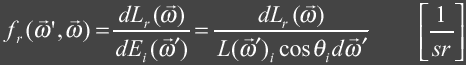where: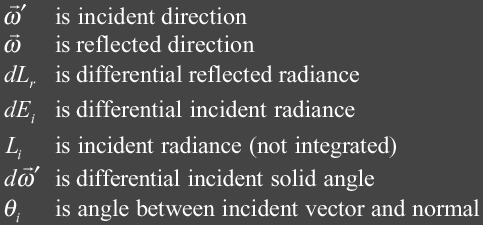For a BRDF to be physically plausible it must follow the law of energy conservation and must obey the Helmholtz reciprocity principle.  Following the law of energy conservation the BRDF should evaluate to a real number in the range [0, 1].  To be more specific the differential BRDF integrated over a hemisphere must be less then or equal to one.  This means we can not reflect more light then we have received.  This can be expressed mathematically as follows: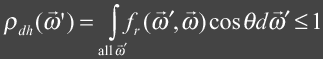This expression is also known as the directional hemispherical reflectance and describes the total amount of incident light energy that is reflected which must be less then or equal to 1.  Helmholtz reciprocity principle means that the sampling incident and reflected directions of the BRDF can be switched and the result will be the same.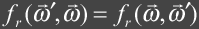Obtaining a BRDF for a material can be done in several ways.  One can obtain a BRDF through empirical measurements and then fit a mathematical function to the data.  Some examples of these empirical models are the popular Lambert, Phong, Blinn-Phong and Ward models.  The BRDF for a perfectly lambertian (diffuse) surface is just a constant as light is equally reflected in all directions.  Physically based models can be developed analytically and are rooted in physics.  Examples of these are Cook-Torrance model and a model presented by Kajiya in 1985 that handles anisotropic reflection.

## Measuring and Storing BRDFs

Several difficulties arise when attempting to measure BRDFs.  The first has to do with the precision of the equipment.  In addition we can only measure a finite set of samples.  Due to this the measurements tend to be noisy and additional techniques must be employed to compensate.

Measuring the BRDF over the hemisphere for an opaque surface generates a large amount of data.  For translucent we are dealing with measurements over the sphere.  Finding a good compression technique would be highly desired.  In addition, since we are measuring only finite quantities we would like to find a techniques for finding the BRDF values in between measured samples.

There are several techniques used to store BRDF measurements.

• Uniform and Non-uniform grid
This naive approach stores the sample points directly as a grid.  To obtain the BRDF values between sample points neighboring sample points much be interpolated.  One approach uses quadrilinear interpolation in which the 4 neighboring sample points are interpolated.  This approach doesn't provide tangential continuity.  Also, interpolation for non-uniform grids are more difficult.
• Interpolating Spline
Fits an interpolating spline through the sample points.  This approach provides smooth interpolation between sample points but often introduces high frequency noise due to oscillations in the curve.  The noise shows up as artifacts in rendering.
• B-Spline
Uses the sample points as control points for the spline.  It provides smooth interpolation but the BRDF values at the sample points are not the original measured values.
• Spherical Harmonics
This common technique represents BRDFs as a infinite sum of spherical harmonic basis functions.  Large number of terms are needed to accurately represent general BRDFs and this technique also introduces high frequency noise.  There are several techniques for noise reduction that are used.
• Wavelets
Wavelets are basis functions that are good at representing signals that contain a great deal of high frequency signals in some areas while little in others.  Wavelets are very attractive for storing BRDFs for this reason.  Wavelets also provide good compression while introducing little error.

A gonioreflectometer is a device for measuring BRDFs.  It usually consists of a light source and a detector that can be controlled to include the necessary four degrees of freedom.  Physical gonioreflectometer implementations typically fall into two categories: traditional and image-based.  In addition to these, virtual gonioreflectometers obtain BRDFs through simulation.

The figure below shows a traditional gonioreflectometer designed by Murray-Coleman and Smith.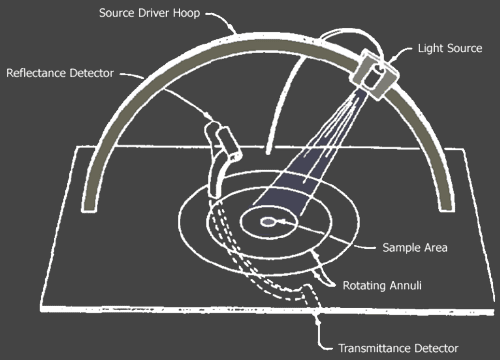Murray-Coleman and Smith Gonioreflectometer. ( Figure copied and modified from Ward )

It has a lamp attached to a motorized carriage that moves along a hoop which is used to control the incident illumination angles.  The light source is an incandescent lamp that is fixed below the table.  A fibre-optic cable routes the light up to the lamp.  The luminance can be adjusted according to the reflectance of the sample.  It also has a detector that is mounted to a ring-like structure called an annuli that control the reflection angles for measurement.  The optical arrangement of the lens and aperture restricts the field of view of the detector to the sample and nearby area only.

## Imaging Gonioreflectometer

In the same paper that Ward introduces a new shading model he describes a new imaging gonioreflectometer developed at the Lighting Systems Research Group at Lawrence Berkeley Laboratory.  This device is designed to capture an entire hemisphere of reflected light at once.  Capturing BRDF with this device is much faster then that of traditional gonioreflectometers.  Ward states that a complete BRDF can be recorded in a matter of minutes which includes the time for manually rotating the sample.  In addition it can capture anisotropic reflectance distribution.  The figure below shows the setup.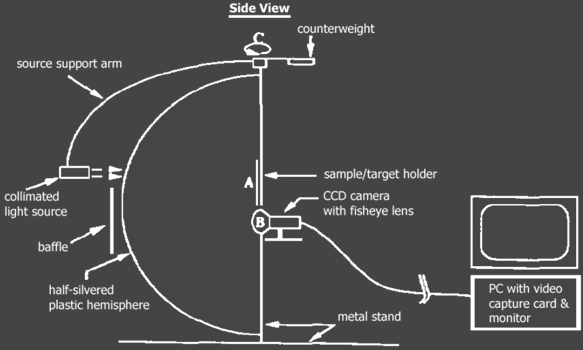It employs a CCD camera fitted with a fish-eye lens to measure reflectance gathered by a half-silvered mirror from a target sample.  The light source is collimated, meaning that it is designed so that the light rays are made parallel, and is setup on a arm outside the hemisphere.  The hemisphere allows light through to the target sample.  One benefit of this arrangement, that Ward states in , is that this setup allows for the measurement of retroreflection where the light is reflected back towards the light source.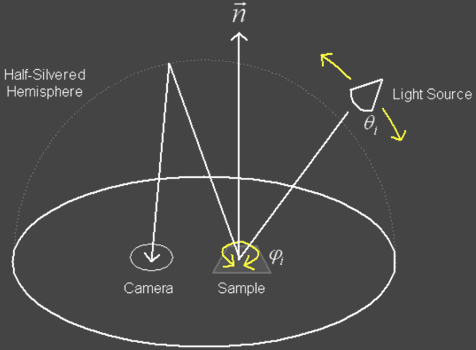The incident angles for measurement can be adjusted by rotating the target sample and moving the light source arm.  These are mechanically controlled by a computer.

A small disadvantage with this device is that it can not capture reflectance at near grazing angles.  It also is unable to measure polished surfaces with sharp specular peaks, due to the optical precision of the hemisphere.  Technically the hemisphere should be a hemi-ellipsoid.

Ward proposes a simple empirically based mathematical shading model that is easy to implement.  It has a few simple parameters to help fit the function to measured BRDF data.  The equation is based on a Gaussian model of slope distributions used to describe the roughness of a surface.

It is know in physics that rough surfaces tend to have Gaussian or normal distributions.  It is the law of large numbers.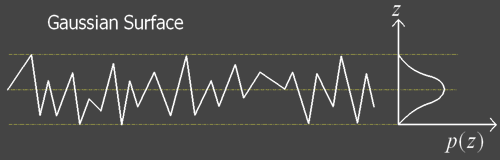Figure copied and modified from Lecture 4 of Stanford Lecture Series. 

The figure above shows the Gaussian height distribution for a rough surface.  The distribution curve for a perfectly smooth surface is just a Dirac Delta function or impulse function.  The wider and flatter the bell shape the rougher the surface tends to be.  In other words with a flatter curve the height tends to deviate more.  Ward's model uses a Gaussian model that describes the slope distribution of micro-facets.  Rough surfaces tend to have micro-facets with steeper slopes.

Using slope distributions to describe microfacets was not new.  Cook-Torrance also used slope distributions to describe the roughness of a surface.  The other main components that Cook-Torrance accounted for were a geometric and Fresnel term.  The geometric term describes the self-shadowing effects of the surface.  The Fresnel term describes the amount of light reflected and refracted by the surface.  Ward states that the Fresnel and geometric terms are hard to integrate and often times cancel each other out and he therefore does not include these to keep his model simple.

Ward first describes a mathematical model for isotropic surfaces.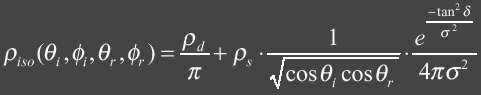where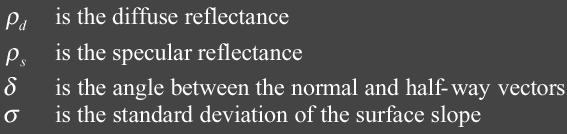The equation has two terms: diffuse and specular.  Its easy to see that the equation is symmetric with respect to its incident and reflected angles as required for a physically plausible BRDF.  The centerpiece of this equation is the exponential and is used to describe the shape distribution of the specular lobe.  It is normalized by the 1 / 4 * PI * Sigma.  Sigma is the parameter that controls the shape and is a term that can be measured from real-world isotropic surfaces.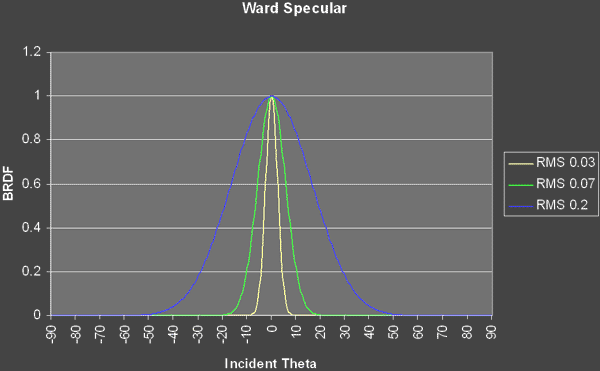The figure above shows plots of the BRDF specular component for RMS values of 0.03, 0.07 and 0.2.  For this plot the incident illumination angles are ( 0, 0 )

Ward's anisotropic model is an extension of the isotropic model that handles varying slope distributions in two perpendicular directions.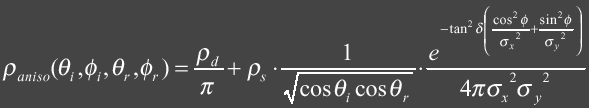where: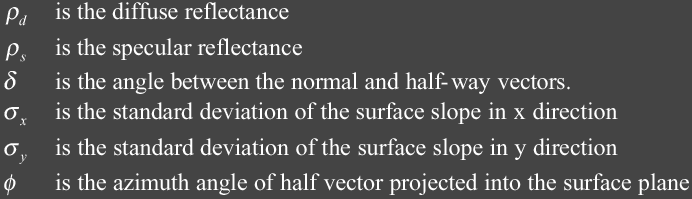This version has four controllable terms and Ward shows that these terms have physical meaning as long as the sum of the diffuse and specular terms are less then 1 and the two sigma terms are not too large.  The figure below shows the geometry for anisotropic surface.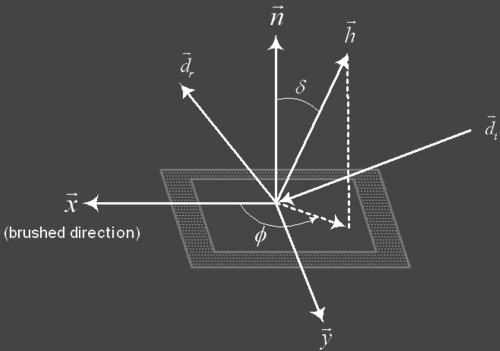Ward discusses using a combination of deterministic and stochastic sampling techniques for implementing the rendering equation.  It be describe as: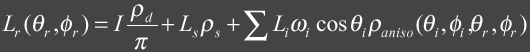where: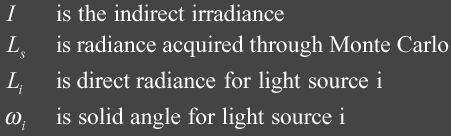The Ls term is computed by using Monte Carlo to sample the hemisphere above the surface where the specular lobe lies.

## Bidirectional Texture Function

Dana et al. describe a method for capturing variation of a textured surface with illumination and viewing directions.  The device captures images of a textured surface with varying light and viewing position.  The images are compiled into a database and a function is used to query into it.  The database and the function is known as a bidirectional texture function ( BTF ).  BTFs are useful for describing fine scale appearances of surfaces.

A BTF is a 6D function of 2D position, viewing and illumination angles.  The BTF can be treated as a single texture map where the values of the texels are a 4D function of viewing and lighting directions.  The u and v coordinates used to index the map is the position.  Since the BTF consists of a large amount of images of the texture, computing the lookup value at (u,v) is expensive.  It requires the lookup of all the samples that meet the criteria as specified by the lighting and viewing directions.  To compute the final texture color these samples are then blended together.  Overlapping regions of the samples are blended together.

The device for generating a BTFs has a CCD color video camera, robot arm for orienting samples, light source with fresnel lens, spectrometer and PC for capturing the images.  The fresnel lens is used to collimate the light.  For BTF measurements the light source is fixed and the camera is positioned at seven locations.  For each of the camera location images are captured for various orientations of the texture sample where it is both visible and illuminated.  In the figure below the vertices of the hemisphere correspond to the direction of the sample's surface normal.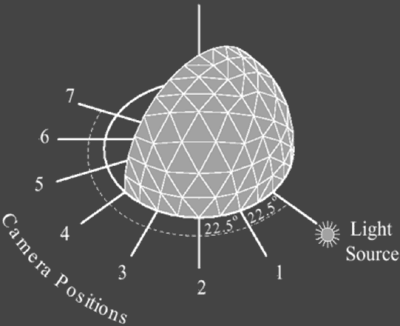Image copied and modified from 

When the device is capturing the BTF for surface it is also capturing the BRDF of the surface.  Each BTF sample is a slice of the BRDF.  The following image from Dana et al. shows the relationship.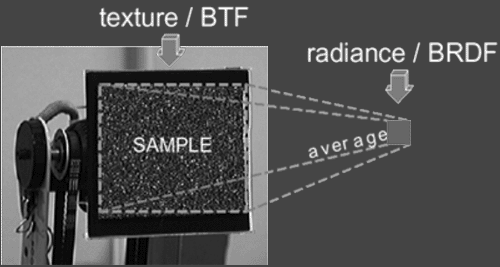The figure below can show the fine detail that can be captured by BTFs.  The cylinder on the left is texture mapped with one captured sample while the image on the right is mapped with a BTF.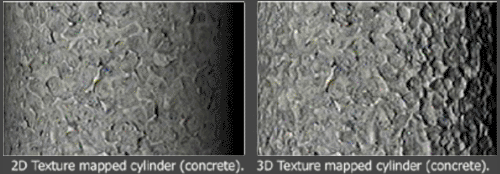Image is copied from 

The bunnies in the picture below is another example of the difference between standard texture mapping and BTF surface mapping.  The same BTF was used for all bunnies.  The only difference is the light position used in which the two bunnies on the left used a different lighting setup then the two bunnies on the right.  In the right corner of the picture shows a slice of the BTF map that was used.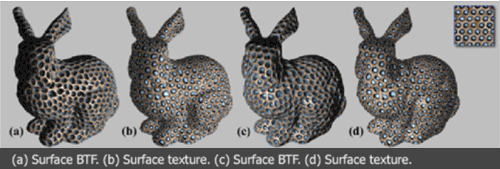Image is copied from [

## References

  Ward, Measuring and Modeling Anisotropic Relfection, Proc. ACM SIGGRAPH 1992  White D.R. et al., Reflectometer for Measuring the Bidirectional Reflectance of Rough Surface, Applied Optics 37:16, 1998  Dana et al, Reflectance and Texture of Real-World Surfaces, ACM Transactions of Graphics 18:1, 1999  Tong et al., Synthesis of Bidirectional Texture Functions on Arbitrary Surfaces, ACM SIGGRAPH 2002  Ke, A Method of Light Refletance, Masters Thesis, University of British Columbia, 1999  DeYoung, Properties of Tabulated Bidirectional Reflectance Distribution Functions, Masters Thesis, University of British Columbia, 1996  Glassner, Andrew Principles of Digital Image Synthesis, Morgan Kaufmann Publishers, Inc., San Fransico, 1995  Shirley, Peter Fundamentals of Computer Graphics, A K Peters, Ltd, Massachusetts, 2002  Hanrahan et al., Appearance Models for Computer Graphics and Vision, Stanford Lecture Notes at http://graphics.stanford.edu/courses/cs448c-00-fall/, 2000